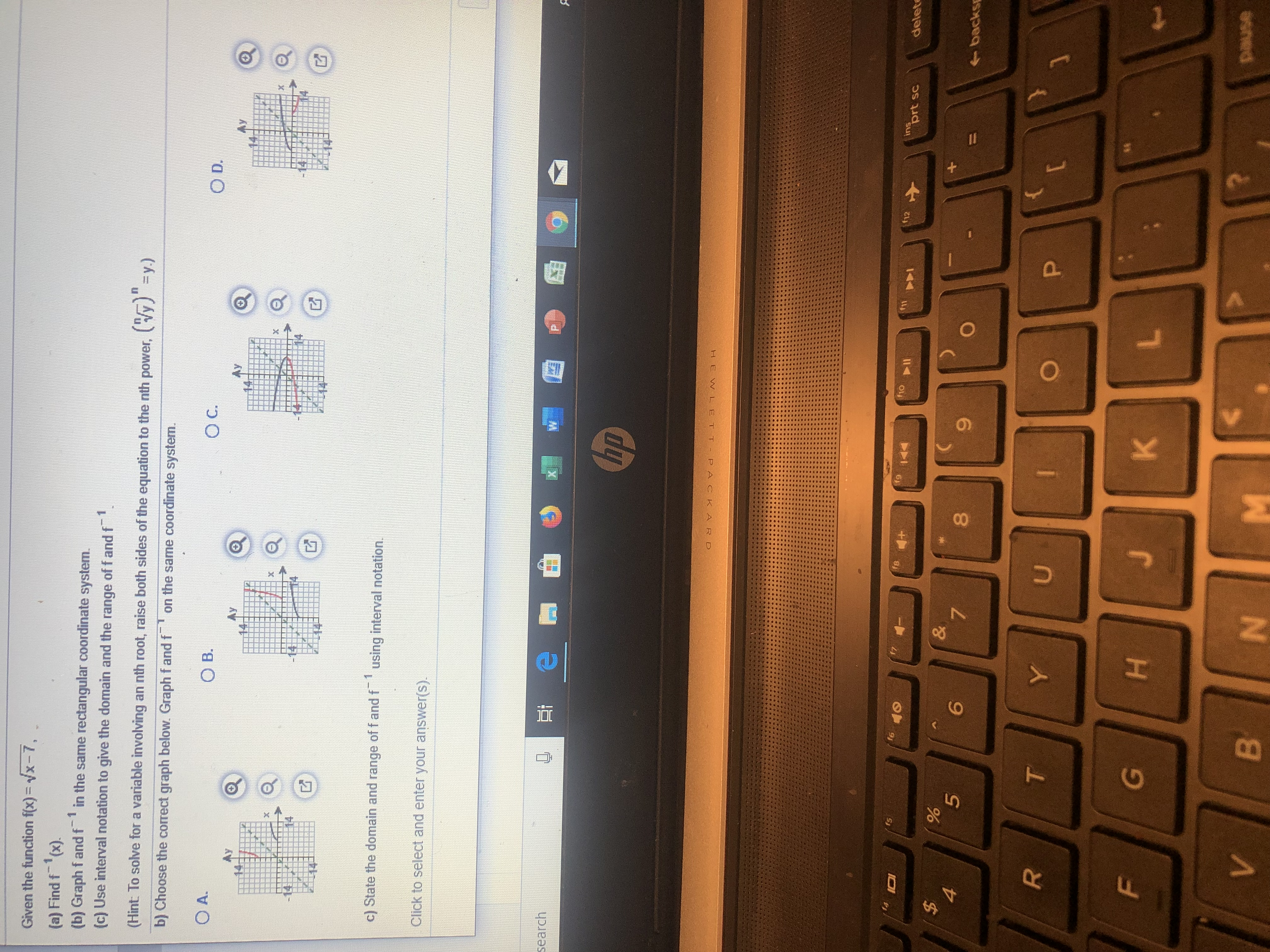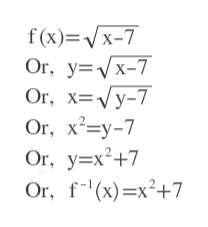Given the function f(x) = vx-7,(a) Find f (x)(b) Graph f and f(c) Use interval notation to give the domain and the range off and fin the same rectangular coordinate system.1Fy.)an nth root, raise both sides of the equation to the nth power(Hint To solve for a variable involvingb) Choose the correct graph below. Graph f and f on the same coordinate system.O D.O C.O B.O A.AyAyAy1using interval notation.c) State the domain and range of f and fClick to select and enter your answer(s).XsearchhpHEWL E T T P ACKA RDinsprt scf12f6f11f5deleteI0I&7+56backsTYUFGKpauseBVM1OO96LL

Questionhelp_outlineImage TranscriptioncloseGiven the function f(x) = vx-7, (a) Find f (x) (b) Graph f and f (c) Use interval notation to give the domain and the range off and f in the same rectangular coordinate system. 1 Fy.) an nth root, raise both sides of the equation to the nth power (Hint To solve for a variable involving b) Choose the correct graph below. Graph f and f on the same coordinate system. O D. O C. O B. O A. Ay Ay Ay 1 using interval notation. c) State the domain and range of f and f Click to select and enter your answer(s). X search hp HEWL E T T P ACKA RD ins prt sc f12 f6 f11 f5 delete I0I & 7 + 5 6 backs T Y U F G K pause B V M 1 OO 96 LL fullscreen
Step 1

We first replace f(x) by y. Then we switch x and y and solve for y. This new y is replaced by f^-1(x).help_outlineImage Transcriptionclosef(x) Vx-7 Or, y x-7 Or, х%3DVу-7 Or, xy-7 Or, y x2+7 Оr, f(x) —x?+7 fullscreen
Step 2

Want to see the full answer?

See Solution

Want to see this answer and more?

Our solutions are written by experts, many with advanced degrees, and available 24/7

See Solution
Tagged in

Other